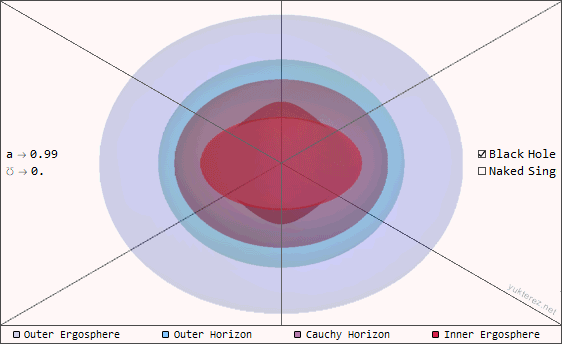## Naked Singularities

English Version
Yukterez
Beiträge: 269
Registriert: Mi 21. Okt 2015, 02:16

### Naked SingularitiesAlthough naked singularities might not exist in nature, General Relativity allows them so they are still of at least theoretical interest. The images and videos show the gravitational lensing of electrically overcharged Reissner Nordström, overextremal Kerr and also charged and spinning Kerr Newman singularities. The raytracing was done with the Yukterez Raytracer, which is written in Mathematica Syntax.The total energy Mc² of the naked singularities on this page is the same in all examples, but its irreducible mass (ℳ), electric charge (℧) and angular momentum (a) components are split up differently. The following animation shows a naked singularity with different inclinations (0°=polar, 90°=equatorial).Kerr Newman, M=1, a=1, ℧=1 → ℳ=½√(1+2i) - r=50GM/c², θ=0°..90°, FOV=120°×60° (click on the gif to load a higher resolution mp4):Background: Milky Way panorama (ESO/Brunier), unlensed view with FOV=120°×60°:Gravitational lense of a naked singularity with a=1.5, ℧=0.4 in the vicinity of the earth, ZAMO perspective (click to play animation):Accretion disk around a naked singularity with a=1, ℧=0.3, inner radius: ri=1, outer radius: ra=10:Simon Tyran @ minds || gab || wikipedia || stackexchange || wolframYukterez
Beiträge: 269
Registriert: Mi 21. Okt 2015, 02:16

### Naked SingularitiesImages (a²+℧²=2², click to enlarge)Reissner-Nordström: M=1, a=0, ℧=2 → ℳ=½+i√¾ - Observer: r=50GM/c², θ=egal (symmetric), FOV=120°×60°:Kerr-Newman: M=1, a=√2, ℧=√2 → ℳ=∜(3/16)·(1+i) - Observer: r=50GM/c², θ=90° (equatorial view), FOV=120°×60°:Kerr-Newman: M=1, a=√2, ℧=√2 → ℳ=∜(3/16)·(1+i) - Observer: r=50GM/c², θ=45° (inclined view), FOV=120°×60°:Kerr-Newman: M=1, a=√2, ℧=√2 → ℳ=∜(3/16)·(1+i) - Observer: r=50GM/c², θ=1° (polar view), FOV=120°×60°:Kerr: M=1, a=2, ℧=0 → ℳ=√¾+i/2 - Observer: r=50GM/c², θ=90° (equatorial view), FOV=120°×60°:Kerr: M=1, a=2, ℧=0 → ℳ=√¾+i/2 - Observer: r=50GM/c², θ=45° (inclined view), FOV=120°×60°:Kerr: M=1, a=2, ℧=0 → ℳ=√¾+i/2 - Observer: r=50GM/c², θ=1° (polar view), FOV=120°×60°:Further reading and comparison: Waseda, p.14 & DeVries, p.20Simon Tyran @ minds || gab || wikipedia || stackexchange || wolframYukterez
Beiträge: 269
Registriert: Mi 21. Okt 2015, 02:16

### Naked SingularitiesVideos: equatorial orbits, φ=0..360° (a²+℧²=1.01²)Reissner Nordström, M=1, a=0, ℧=1.01 → ℳ=½+0.0708872i - r=20GM/c², θ=90°, FOV=154.8°×77.4°:Kerr, M=1, a=1.01, ℧=0 → ℳ=0.708872+0.05i - r=20GM/c², θ=90°, FOV=154.8°×77.4°:Kerr Newman, M=1, a²+℧²=1.01² → ℳ=0.61305+0.0578152i - r=20GM/c², θ=90°, FOV=154.8°×77.4°:Full HD videos: raytracing.yukterez.netSimon Tyran @ minds || gab || wikipedia || stackexchange || wolframYukterez
Beiträge: 269
Registriert: Mi 21. Okt 2015, 02:16

### Naked SingularitiesHorizons and ergosurfaces for different spin and charge parameters:Surface plot in cartesian coordinates (these surfaces would not be directly visible). Naked singularities would not have inner and outer horizons, only inner and outer ergospheres. Units: G=M=c=K=1:Free fall of a neutral particle on to a charged and rotating (℧=0.4, a=1.5) naked singularity:Geodesic orbit around a naked singularity (℧=0.4, a=1.5):Photon orbit around a rotating (a=1.5) naked singularity:Photon orbit around a rotating (a=0.9) and charged (℧=0.9) naked singularity:Polar plunge ping pong of a particle above a rotating (a=1.5) naked singularity:Particle orbit around a rotating (a=1.25) naked singularity:Escape from a rotating (a=2) naked singularity:0 energy retrograde stationary photon orbit (a=0.5, ℧=1), ergoring instead of ergospheres:Retrograde equatorial photon orbit (a=0.5, ℧=1), radial turning points at r=0.8 and r=1.3484:Click on the images to play the animations. Code: kerr.newman.yukterez.net and raytracing.yukterez.netAll work here as well as the 3rd party source material (the raw panorama photo) is published under the Creative Commons License. Simon Tyran (Yukterez), 2018Simon Tyran @ minds || gab || wikipedia || stackexchange || wolfram